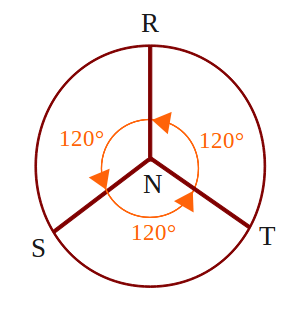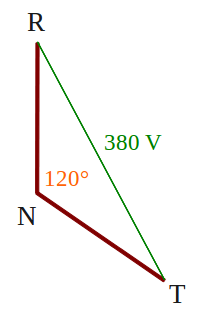# How Voltage Three-phase 380 V Can Be a Single-phase 220 V?

3-phase electrical voltage that usually denoted by R - S - T wire, when measured with a volt meter, inter-phase (RS, or RT, or ST) will show the number 380 V. But when each phase is connected with the neutral wire (RN, or SN, or TN), will show number 220 V. How could that happen?

On the power grid 380 V 3 phase, that distinguishes the phase R - S - T is phase angle, which is odds of 120 °. When we have described, it would look like thisBy taking one of the blade, it would look like the following pictureWe use the triangle rule, where the number of angles in a triangle is equal to 180 °, obtained angle RTN and TRN, respectively (180 ° -120 °) / 2 = 30 °After that we use the sine rule,
380 V / sin 120 ° = RN / sin 30 °
380 V sin 30 ° = sin 120 ° RN
190 V = 0.886 RN
RN = 190 V / 0.866
RN = 220 V

That's it, voltage 3 phase 380 V can be a 1 phase 220 V, mathematics is magic.

Next→
←Prev

### Choose label

1.2.3.